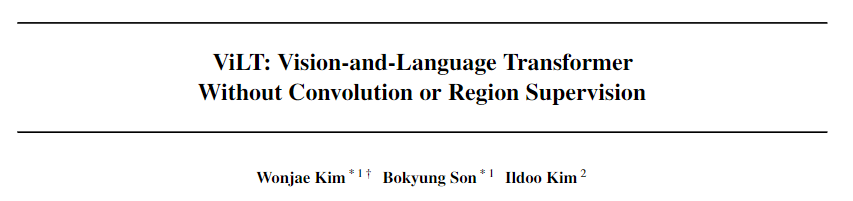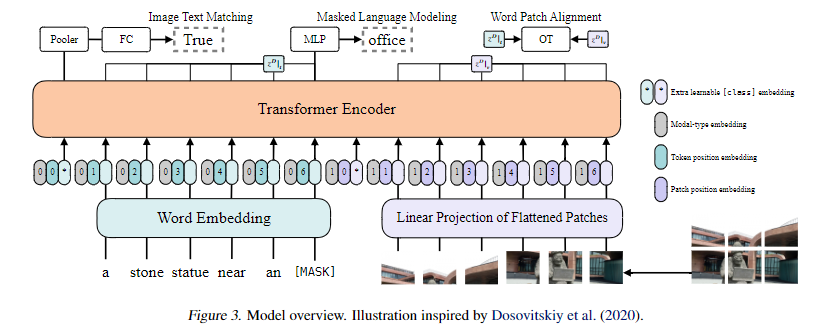V1

2022/12/09阅读：85主题：默认主题

# 图文结合-ViLTViLT: Vision-and-Language Transformer Without Convolution or Region Supervision

https://arxiv.org/abs/2102.03334

https://github.com/dandelin/vilt

ViLT以一种统一的方式处理两种模态的信息。其优势在于：

• 使用transformer处理视觉特征，丢弃了原始的单独处理视觉特征的模块，显著优化了运行时间
• 单流模型，使用基于多层transformer结构的交互层，实现不同模态的深层交互

ViLT是一个单流模型，作者认为双流模型引入了额外参数。在处理视觉特征上，ViLT使用patch projection的方式来代替区域或网格特征。

#### 1、模型结构

ViLT的模型结构如下：从图中可以看到，文本用0表示，图像用1表示，然后根据各自的位置得到其位置ids，并且，文本的预处理仅一个word embedding，图像的预处理也仅有分patch和linear projection（更具体地，其实是一个卷积层，不过卷积本来也是线性变换，所以说线性层也不错）。最后将concat的向量输入交互层，通过transformer实现模态信息的深层交互。

class PatchEmbed(nn.Module):    """ Image to Patch Embedding"""    def __init__(        self,        img_size=224,        patch_size=16,        in_chans=3,        embed_dim=768,        no_patch_embed_bias=False,    ):        super().__init__()        img_size = to_2tuple(img_size)        patch_size = to_2tuple(patch_size)        num_patches = (img_size // patch_size) * (img_size // patch_size)        self.img_size = img_size        self.patch_size = patch_size        self.num_patches = num_patches        self.proj = nn.Conv2d(            in_chans,            embed_dim,            kernel_size=patch_size,            stride=patch_size,            bias=False if no_patch_embed_bias else True,        )    def forward(self, x):        B, C, H, W = x.shape        # FIXME look at relaxing size constraints        x = self.proj(x)        return x

transformer模态交互层，一层的代码如下：

class Block(nn.Module):    def __init__(        self,        dim,        num_heads,        mlp_ratio=4.0,        qkv_bias=False,        qk_scale=None,        drop=0.0,        attn_drop=0.0,        drop_path=0.0,        act_layer=nn.GELU,        norm_layer=nn.LayerNorm,    ):        super().__init__()        self.norm1 = norm_layer(dim)        self.attn = Attention(            dim,            num_heads=num_heads,            qkv_bias=qkv_bias,            qk_scale=qk_scale,            attn_drop=attn_drop,            proj_drop=drop,        )        # NOTE: drop path for stochastic depth, we shall see if this is better than dropout here        self.drop_path = DropPath(drop_path) if drop_path > 0.0 else nn.Identity()        self.norm2 = norm_layer(dim)        mlp_hidden_dim = int(dim * mlp_ratio)        self.mlp = Mlp(            in_features=dim,            hidden_features=mlp_hidden_dim,            act_layer=act_layer,            drop=drop,        )    def forward(self, x, mask=None):        _x, attn = self.attn(self.norm1(x), mask=mask)        x = x + self.drop_path(_x)        x = x + self.drop_path(self.mlp(self.norm2(x)))        return x, attn

forward的计算过程中可以看出，确实是先进行LN然后进行attn或mlp，其他的结构与bert是一致的，都是12层。

#### 2、预训练任务

ViLT使用的是图文结合常规的预训练任务，ITM和MLM。

（1）ITM

（2）MLM

RandAugment的实现如下：

class RandAugment:    def __init__(self, n, m):        self.n = n        self.m = m  # [0, 30]        self.augment_list = augment_list()    def __call__(self, img):        ops = random.choices(self.augment_list, k=self.n)        for op, minval, maxval in ops:            val = (float(self.m) / 30) * float(maxval - minval) + minval            img = op(img, val)        return img

#### 3、下游任务

ViLT的下游任务有两类，分类和检索。其中分类包括VQA和NLVR，检索就是ITM。

（1）分类

（2）检索

ViLT与 SOHO 有着共同的目的，也就是去掉视觉编码器中的rigion提取结构，以降低模型在推理的延时。但是，ViLT是单流的方式，相比SOHO实现了模态之间更深层次的信息交互，在极快的推理速度下，也保证了在NLVR2等任务上的性能。V1